# Calculate the Area in Excel

In Excel, you may compute the area of a parallelogram by using a conventional formula and the multiplication operator.

To get the area of a circle, use the PI function and the exponent operator (). Excel has a standard set of arithmetic operators for performing addition, subtraction, multiplication, and exponentiation when composing formulae (raising to the power of). Furthermore, Excel has operators for cell ranges, range intersections, and implicit intersections.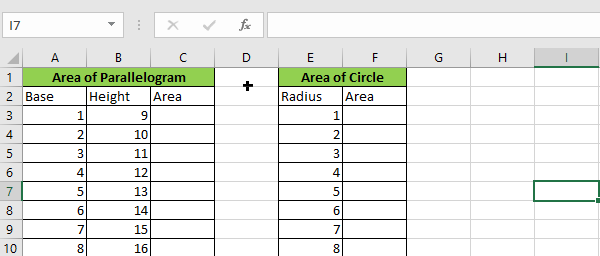## Explanation For Area of a parallelogram in Excel

A parallelogram is a four-sided quadrilateral form having two parallel pairs of sides. A parallelogram’s opposing sides are equal in length, and its opposite angles are equal in size. The area contained by a parallelogram in Euclidean geometry is determined by the formula`A=bh`, where b denotes the base and h denotes the height.

### Parallelogram Formula

As previously stated, a parallelogram is a two-dimensional structure that is studied in geometry. Each figure has its own set of attributes and settings. As a result, certain common formulae are provided for determining these characteristics.

The parallelogram formula is used to calculate both the area and perimeter of a parallelogram.

`A=base*height`

### Area of Parallelogram

The area of a figure is defined as the whole surface area occupied by it, while the area of a parallelogram is defined as the surface area covered or occupied by it. Three distinct techniques are offered for calculating the area of a parallelogram. The area of a parallelogram may be calculated using the figure’s height, side lengths, or diagonals.

The same formula may be expressed in Excel as follows:

`=b*h`

Thus, to get the area of a parallelogram with b equal to 10 and h equal to 20, we may use the following formula:

`=10*20 //it returns 200`

The objective of the example is to determine the area of eleven parallelograms by using the base value in column A and the height value in column B. The formula in C3 is as follows:

`=A3*B3`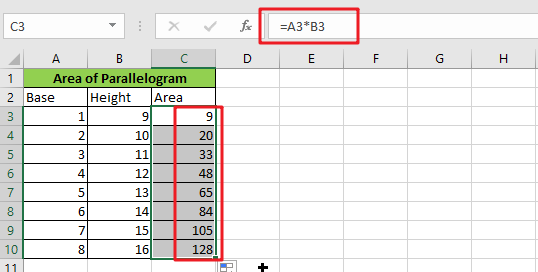Because both cells are entered as relative references, the method generates a different area for each successive row as it is copied along the table.

## Explanation For Area of a circle in Excel

The area contained by a circle of radius (r) is defined in geometry by the following formula: `πr2`

The Greek letter (“pi”) denotes the ratio of a circle’s circumference to its diameter. In Excel, the PI function returns the value 3.141592654, which is accurate to 10 digits:

`=PI() //yields a value of 3.141592654.`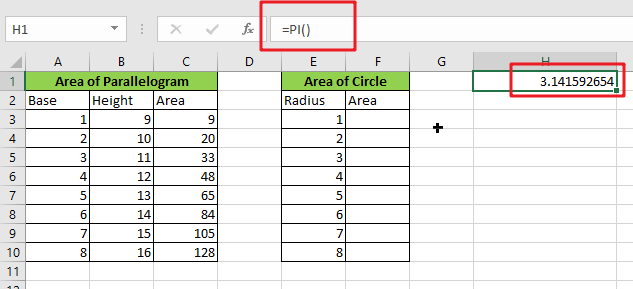In Excel, you may square an integer by using the exponentiation operator ():

`=E3^2`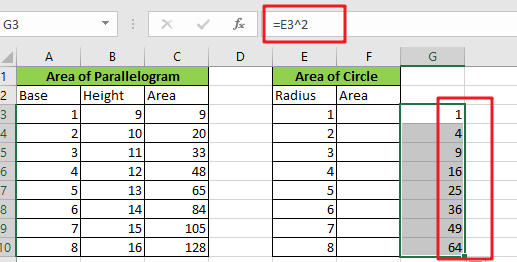Alternatively, you may use the POWER function:

`=POWER(E3,2)`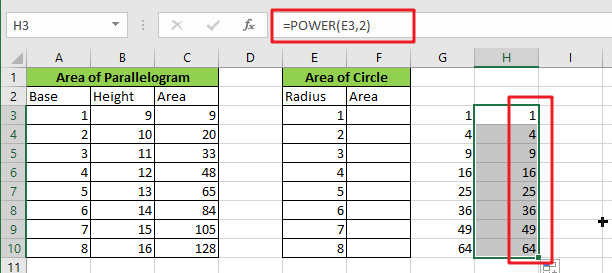By rewriting the formula `=r2 `as an Excel formula, we get the following:

`=PI()*E3^2`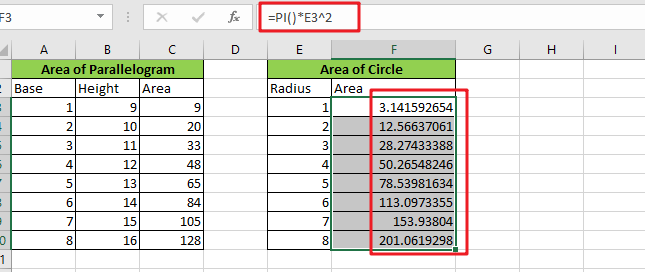Or

`=PI()*POWER(E3,2)`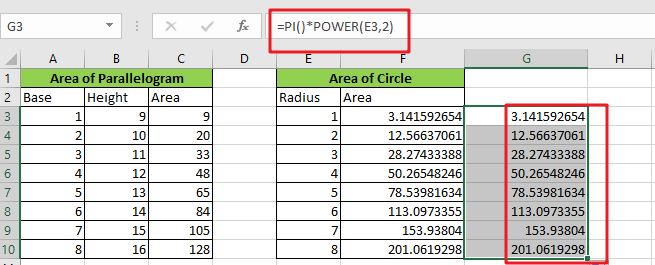Both formulae get the same outcome. Exponentiation occurs before multiplication in Excel’s sequence of operations.

### How It Operates

Pi*Radius2 is the formula for the area of a circle, where Pi is a constant roughly equal to 3.14159265. Excel includes this constant as a function with no parameter inputs called PI ().

The POWER function takes any number and multiplies it by any other number. POWER(Radius,2) returns the Radius’s square. POWER(2,2) returns a value of 4, and 3.14159265*4 equals 12.56637 in our scenario.

### Related Functions

• Excel PI function
The Excel PI function returns the value of mathematical constant PI. The returned value is 3.14159265358979 and ti will accurate to 15 digits.The syntax of the PI function is as below:=PI()…
• Excel POWER function
The Excel POWER function returns the result of a number raised to a given power.The syntax of the POWER function is as below:= POWER (number,power)….